Latest Banking jobs   »

# Quantitative Aptitude Quiz For Bank Foundation 2023 – 4th March

Direction (1-5): The line graph given below shows the production and consumption (in quintal) of Wheat in 5 different years of a village. Study the graph carefully and answer the following questions.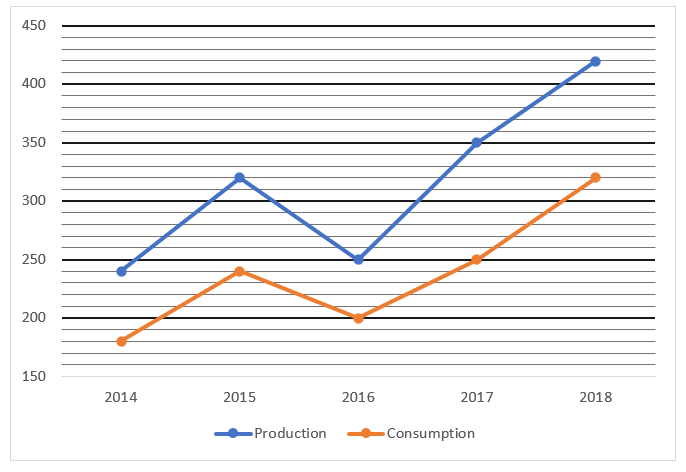Q1. What is the average consumption of Wheat all over the years?
(a) 245 Quintals
(b) 254 Quintals
(c) 316 Quintals
(d) 238 Quintals
(e) 278 Quintals

Q2. Production of wheat in 2015 is what percentage more/less than that in 2016?
(a) 28%
(b) 33%
(c) 30%
(d) 25%
(e) 23%

Q3. What is the ratio between the consumption of wheat in 2016 and 2017 together to the production of wheat in 2014 and 2015 together?
(a) 45 : 53
(b) 45 : 56
(c) 12 : 17
(d) 15 : 17
(e) 30 : 53

Q4. If in 2019 the ratio between the production and consumption of wheat is 7 : 5 and total production of wheat is 280 Quintal, then consumption of wheat in 2019 is how much more/less than previous year?
(a) 150 quintals
(b) 180 quintals
(c) 140 quintals
(d) 120 quintals
(e) 220 quintals

Q5. In which year percentage of consumption of wheat is maximum with respect to production?
(a) 2016
(b) 2018
(c) 2015
(d) 2017
(e) 2014

Directions (6-10): What value should come in place of (?) in the following questions?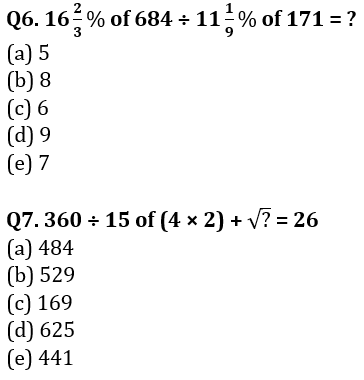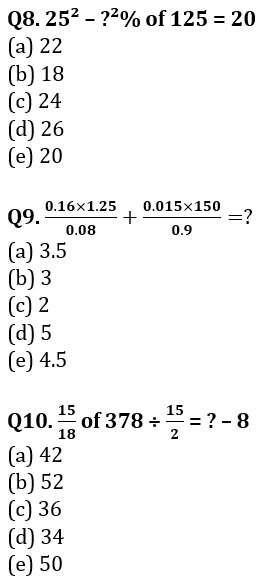Q11. A sum becomes 160% of amount at simple interest in 3 years. Find the compound interest on sum of Rs 12000 at same rate of interest after 2 years.
(a)Rs 4820
(b)Rs 5460
(c)Rs 6280
(d)Rs 5280
(e)Rs 5840

Q12. A bag contains 15 balls numbered from 1 to 15. Two balls are selected randomly without replacement. Find the probability that both balls are even number ball.
(a)1/5
(b)3/5
(c)2/5
(d)4/5
(e)13/15

Q13. A person bought an old bicycle in Rs 6400. He expends Rs. X on repairing it. Find value of X, if he sold the bicycle in Rs 9180 and makes profit of 20%.
(a)Rs 1450
(b)Rs 1250
(c)Rs 1800
(d)Rs 1350
(e)Rs 1150

Q14. In a class of N students, a new student weighing 35 kg joined due to which average weight of the class increased by 1 kg, if the weight of new student would have be15 kg, then the average weight of the class decreases by 1 kg, then find the total no. of students in class initially.
(a)12
(b)7
(c)15
(d)11
(e)9

Q15. Ratio of two numbers is 5 : 7. If 30 is added in each number, the ratio becomes 3 : 4. Find the ratio of the number if 10 is subtracted from each number.
(a)5 : 8
(b)8 : 11
(c)7 : 10
(d)6 : 11
(d)7 : 9

Solutions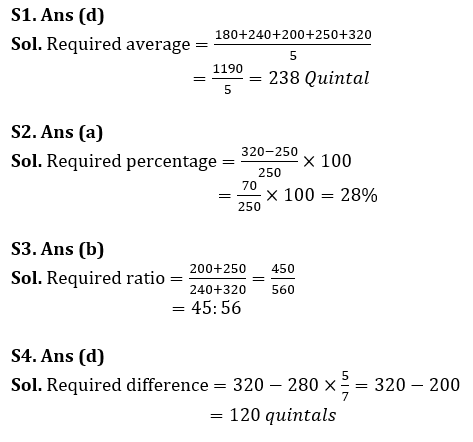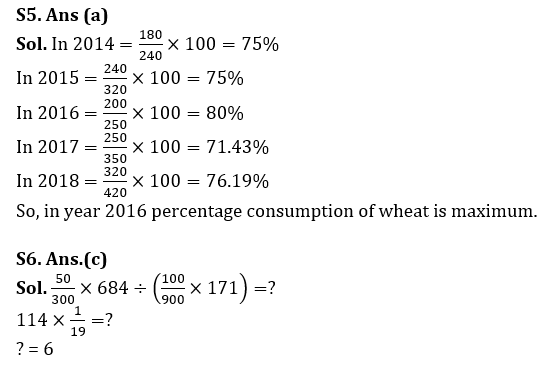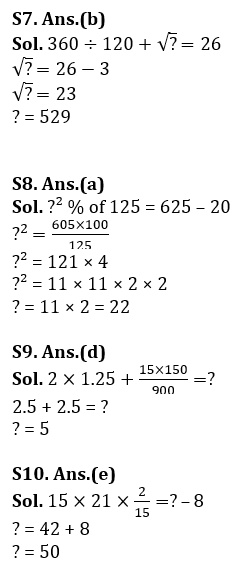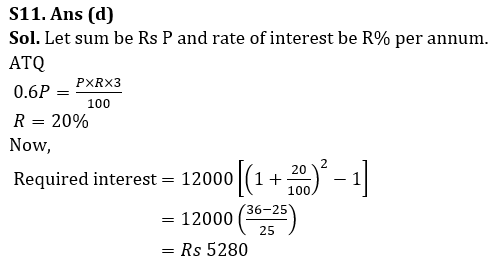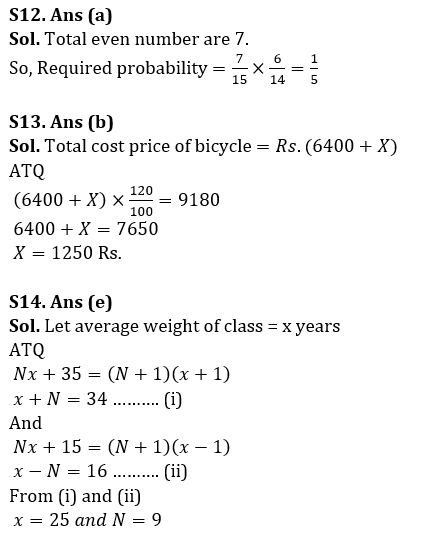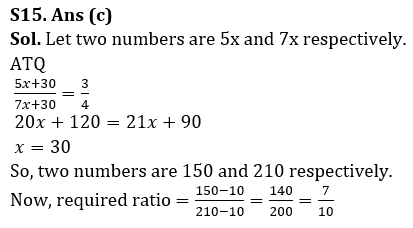.

## FAQs

### What is the selection process of the Bank Clerk?

The selection process of the Bank Clerk is Prelims & Mains.

#### Congratulations!Union Budget 2023-24: Free PDF